# STATS 330 Lecture 9 3102021 330 lecture 9

• Slides: 31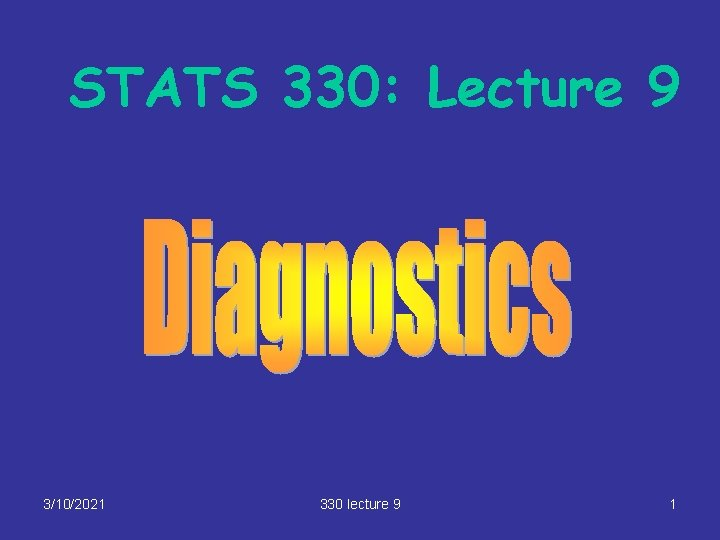STATS 330: Lecture 9 3/10/2021 330 lecture 9 1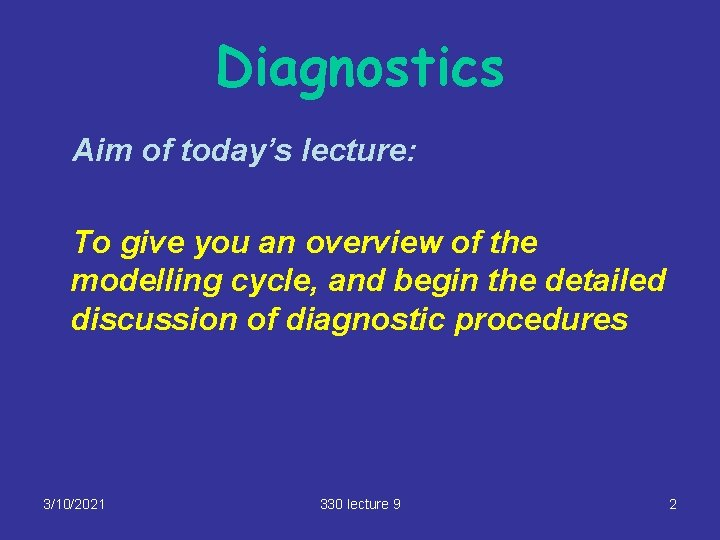Diagnostics Aim of today’s lecture: To give you an overview of the modelling cycle, and begin the detailed discussion of diagnostic procedures 3/10/2021 330 lecture 9 2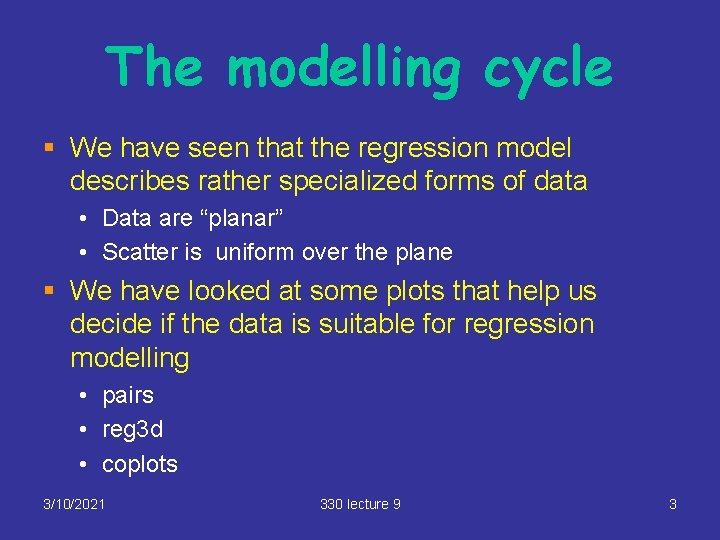The modelling cycle § We have seen that the regression model describes rather specialized forms of data • Data are “planar” • Scatter is uniform over the plane § We have looked at some plots that help us decide if the data is suitable for regression modelling • pairs • reg 3 d • coplots 3/10/2021 330 lecture 9 3Residual analysis § Another approach is to fit the model and examine the residuals § If the model is appropriate the residuals have no pattern § A pattern in the residuals usually indicates that the model is not appropriate § If this is the case we have two options • Option 1: Select another form of model eg non-linear regression – see other courses • Option 2: transform the data so that the regression model fits the transformed data – see next slide 3/10/2021 330 lecture 9 4Modelling cycle This leads us into a modelling cycle • Fit • Examine residuals • Transform data or change model if necessary This cycle is repeated until we are happy with the fitted model Diagramatically…. 3/10/2021 330 lecture 9 5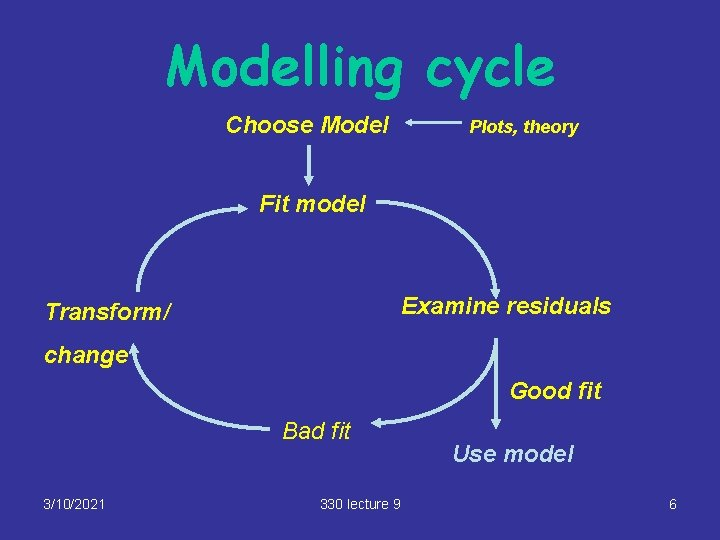Modelling cycle Choose Model Plots, theory Fit model Examine residuals Transform/ change Good fit Bad fit 3/10/2021 330 lecture 9 Use model 6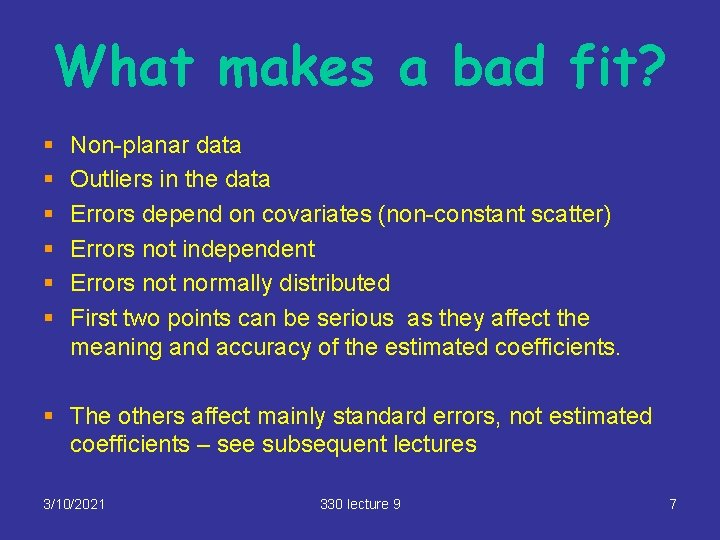What makes a bad fit? § § § Non-planar data Outliers in the data Errors depend on covariates (non-constant scatter) Errors not independent Errors not normally distributed First two points can be serious as they affect the meaning and accuracy of the estimated coefficients. § The others affect mainly standard errors, not estimated coefficients – see subsequent lectures 3/10/2021 330 lecture 9 7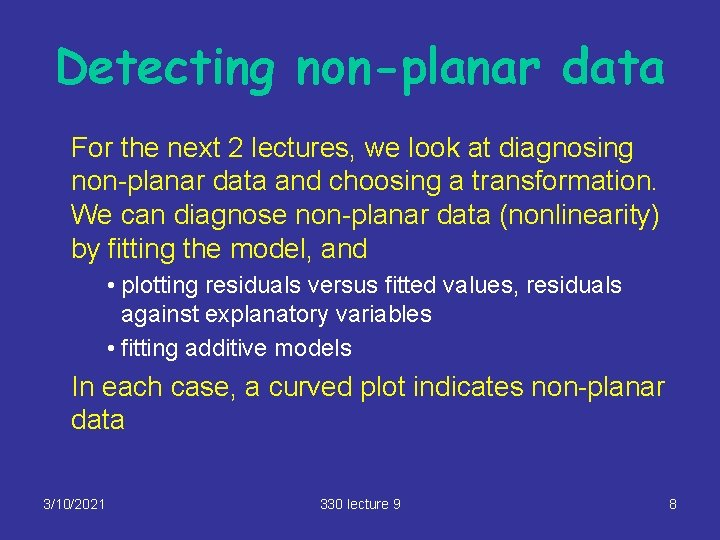Detecting non-planar data For the next 2 lectures, we look at diagnosing non-planar data and choosing a transformation. We can diagnose non-planar data (nonlinearity) by fitting the model, and • plotting residuals versus fitted values, residuals against explanatory variables • fitting additive models In each case, a curved plot indicates non-planar data 3/10/2021 330 lecture 9 8Plotting residuals versus fitted values plot(cherry. lm, which=1) which = 1 selects a plot of residuals versus fitted values 3/10/2021 330 lecture 9 9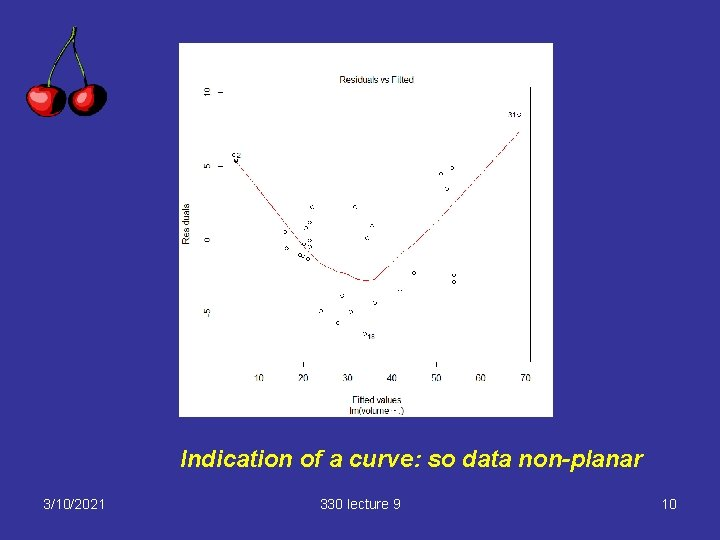Indication of a curve: so data non-planar 3/10/2021 330 lecture 9 10Additive models § These are models of the form Y=g 1(x 1) + g 2(x 2) + … + gk(xk) + e where g 1, … , gk are transformations § Fitted using the gam function in R § The transformations are estimated by the software § Use the function to suggest good transformations 3/10/2021 330 lecture 9 11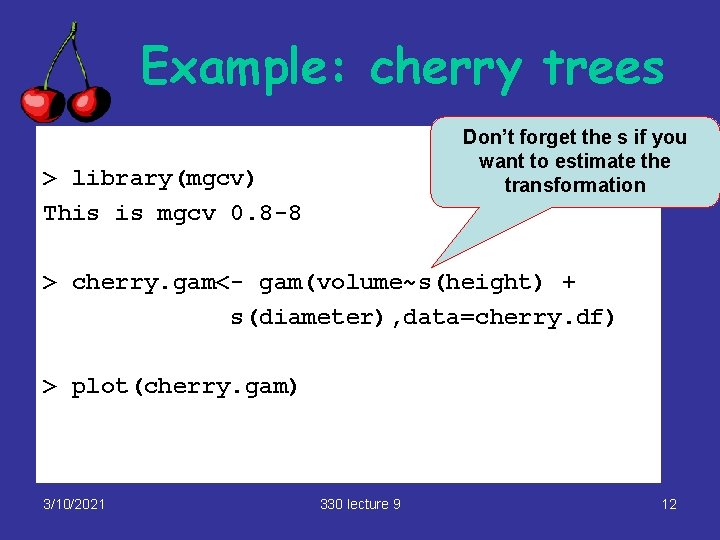Example: cherry trees Don’t forget the s if you want to estimate the transformation > library(mgcv) This is mgcv 0. 8 -8 > cherry. gam<- gam(volume~s(height) + s(diameter), data=cherry. df) > plot(cherry. gam) 3/10/2021 330 lecture 9 12Example Strong suggestion that diameter be transformed 3/10/2021 330 lecture 9 13Fitting polynomials § To fit model y = b 0 +b 1 x + b 2 x 2, use y ~ poly(x, 2) § To fit model y = b 0 +b 1 x + b 2 x 2 + b 3 x 3, use y ~ poly(x, 3) and so on 3/10/2021 330 lecture 9 14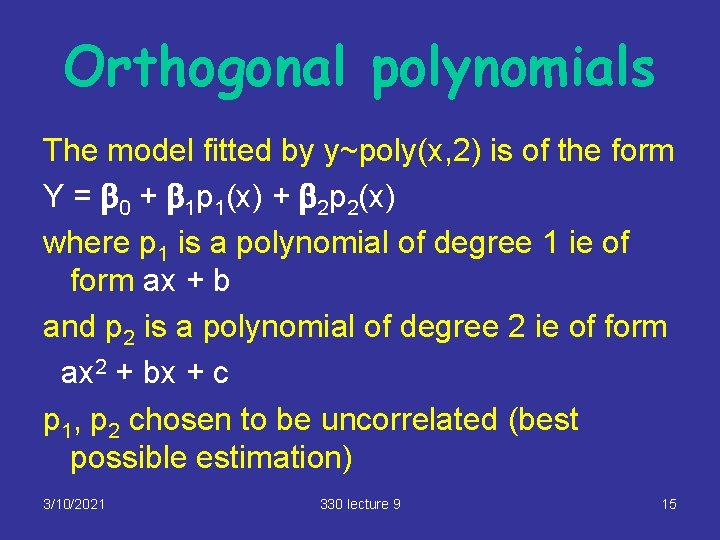Orthogonal polynomials The model fitted by y~poly(x, 2) is of the form Y = b 0 + b 1 p 1(x) + b 2 p 2(x) where p 1 is a polynomial of degree 1 ie of form ax + b and p 2 is a polynomial of degree 2 ie of form ax 2 + bx + c p 1, p 2 chosen to be uncorrelated (best possible estimation) 3/10/2021 330 lecture 9 15Adding a quadratic term (cherry trees) § Suggestion of quadratic behaviour in diameter, so fit a quadratic model in diameter (add a term diameter^2) cherry 2. lm=lm(volume~poly(diameter, 2)+height, data=cherry. df) Coefficients: How to add a quadratic term Estimate Std. Error t value Pr(>|t|) (Intercept) 1. 56553 6. 72218 0. 233 0. 817603 poly(diameter, 2)1 80. 25223 3. 07346 26. 111 < 2 e-16 *** poly(diameter, 2)2 15. 39923 2. 63157 5. 852 3. 13 e-06 *** height 0. 37639 0. 08823 4. 266 0. 000218 *** --Residual standard error: 2. 625 on 27 degrees of freedom Multiple R-Squared: 0. 9771, Adjusted R-squared: 0. 9745 R 2 improved from 94. 8% to 97. 7% 3/10/2021 330 lecture 9 16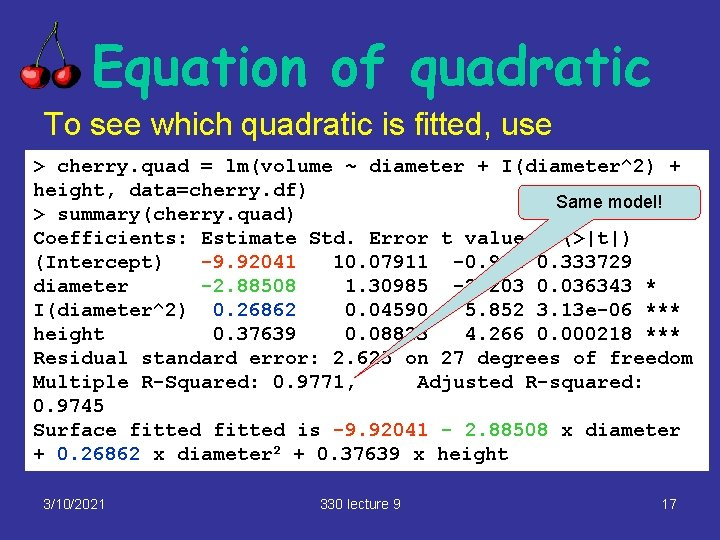Equation of quadratic To see which quadratic is fitted, use > cherry. quad = lm(volume ~ diameter + I(diameter^2) + height, data=cherry. df) Same model! > summary(cherry. quad) Coefficients: Estimate Std. Error t value Pr(>|t|) (Intercept) -9. 92041 10. 07911 -0. 984 0. 333729 diameter -2. 88508 1. 30985 -2. 203 0. 036343 * I(diameter^2) 0. 26862 0. 04590 5. 852 3. 13 e-06 *** height 0. 37639 0. 08823 4. 266 0. 000218 *** Residual standard error: 2. 625 on 27 degrees of freedom Multiple R-Squared: 0. 9771, Adjusted R-squared: 0. 9745 Surface fitted is -9. 92041 - 2. 88508 x diameter + 0. 26862 x diameter 2 + 0. 37639 x height 3/10/2021 330 lecture 9 17Splines § An alternative to polynomials are splines – these are piecewise cubics, which join smoothly at “knots” § Give a more flexible fit to the data § Values at one point not affected by values at distant points, unlike polynomials 3/10/2021 330 lecture 9 18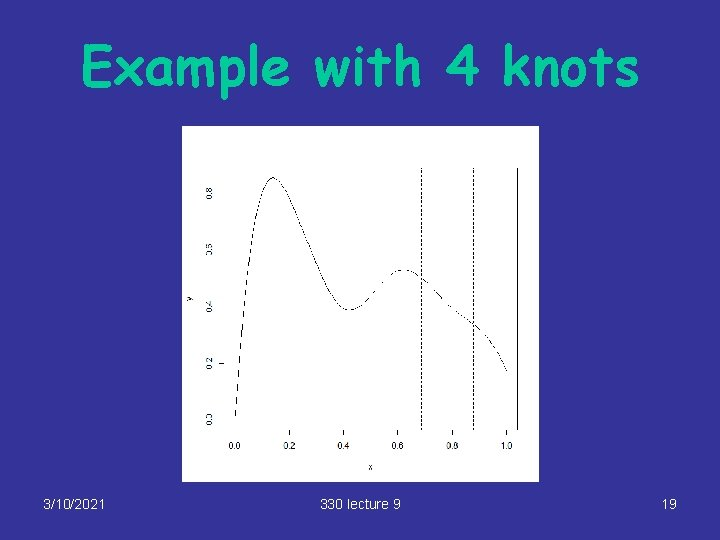Example with 4 knots 3/10/2021 330 lecture 9 19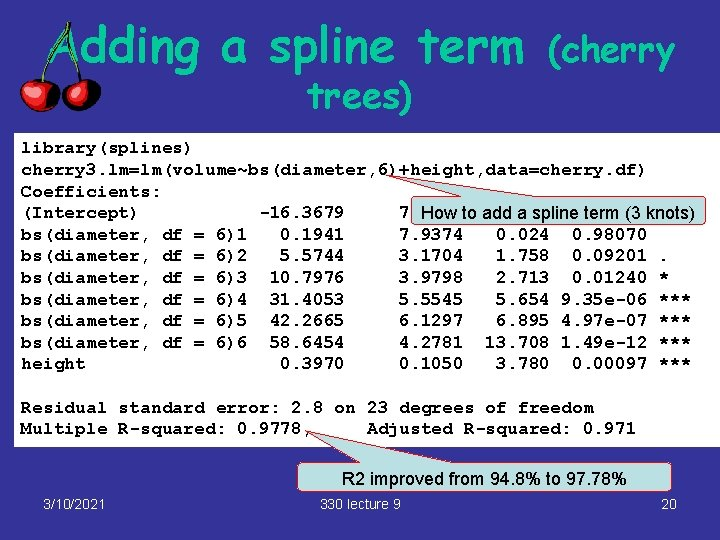Adding a spline term trees) (cherry library(splines) cherry 3. lm=lm(volume~bs(diameter, 6)+height, data=cherry. df) Coefficients: (Intercept) -16. 3679 7. 4856 -2. 187 * How to add a spline 0. 03921 term (3 knots) bs(diameter, df = 6)1 0. 1941 7. 9374 0. 024 0. 98070 bs(diameter, df = 6)2 5. 5744 3. 1704 1. 758 0. 09201. bs(diameter, df = 6)3 10. 7976 3. 9798 2. 713 0. 01240 * bs(diameter, df = 6)4 31. 4053 5. 5545 5. 654 9. 35 e-06 *** bs(diameter, df = 6)5 42. 2665 6. 1297 6. 895 4. 97 e-07 *** bs(diameter, df = 6)6 58. 6454 4. 2781 13. 708 1. 49 e-12 *** height 0. 3970 0. 1050 3. 780 0. 00097 *** Residual standard error: 2. 8 on 23 degrees of freedom Multiple R-squared: 0. 9778, Adjusted R-squared: 0. 971 R 2 improved from 94. 8% to 97. 78% 3/10/2021 330 lecture 9 203/10/2021 330 lecture 9 21Example: Tyre abrasion data Data collected in an experiment to study the abrasion resistance of tyres Variables are Hardness: Hardness of rubber Tensile: Tensile strength of rubber Abrasion Loss: Amount of rubber worn away in a standard test (response) 3/10/2021 330 lecture 9 22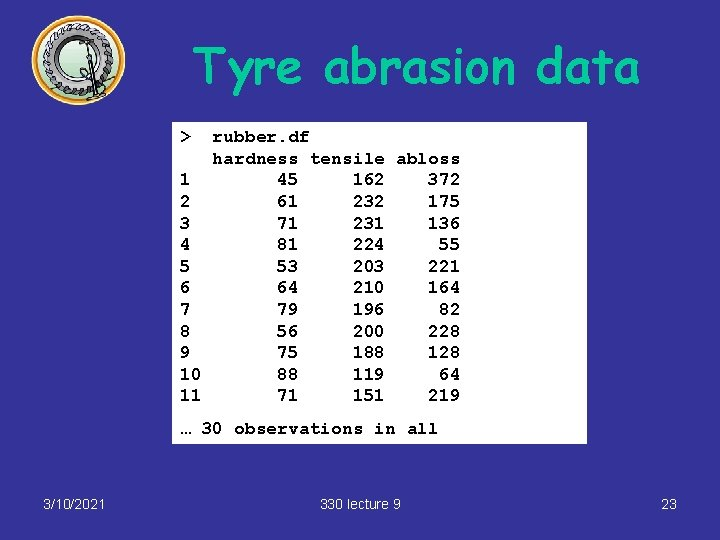Tyre abrasion data > rubber. df hardness tensile abloss 1 45 162 372 2 61 232 175 3 71 231 136 4 81 224 55 5 53 203 221 6 64 210 164 7 79 196 82 8 56 200 228 9 75 188 128 10 88 119 64 11 71 151 219 … 30 observations in all 3/10/2021 330 lecture 9 23Tyre abrasion data > rubber. lm<-lm(abloss~hardness + tensile, data=rubber. df) > summary(rubber. lm) Coefficients: Estimate Std. Error t value Pr(>|t|) (Intercept) 885. 1611 61. 7516 14. 334 3. 84 e-14 *** hardness -6. 5708 0. 5832 -11. 267 1. 03 e-11 *** tensile -1. 3743 0. 1943 -7. 073 1. 32 e-07 *** --Signif. codes: 0 `***' 0. 001 `**' 0. 01 `*' 0. 05 `. ' 0. 1 ` ' 1 Residual standard error: 36. 49 on 27 degrees of freedom Multiple R-Squared: 0. 8402, Adjusted R-squared: 0. 8284 F-statistic: 71 on 2 and 27 DF, p-value: 1. 767 e-11 3/10/2021 330 lecture 9 24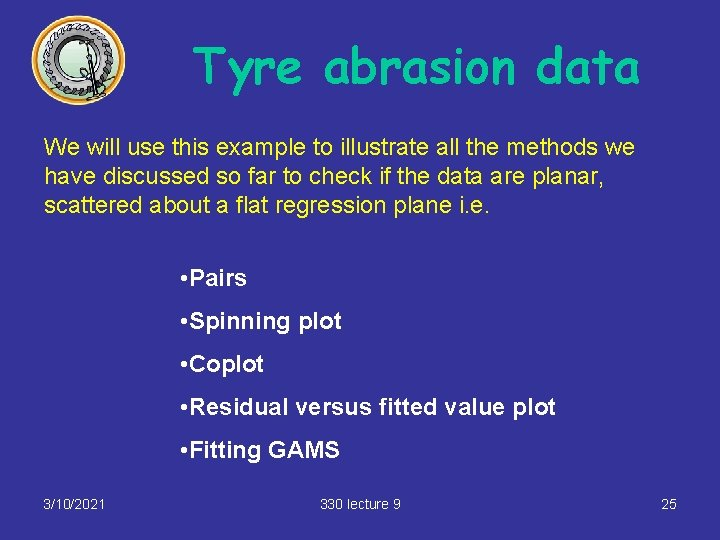Tyre abrasion data We will use this example to illustrate all the methods we have discussed so far to check if the data are planar, scattered about a flat regression plane i. e. • Pairs • Spinning plot • Coplot • Residual versus fitted value plot • Fitting GAMS 3/10/2021 330 lecture 9 25Tyre abrasion data (pairs) 3/10/2021 330 lecture 9 26Spinning 3/10/2021 330 lecture 9 27Tyre abrasion data (coplot) 3/10/2021 330 lecture 9 28Tyre abrasion data: residuals v fitted values data(rubber. df) rubber. lm = lm(abloss~. , data=rubber. df) plot(rubber. lm, which=1) 3/10/2021 330 lecture 9 29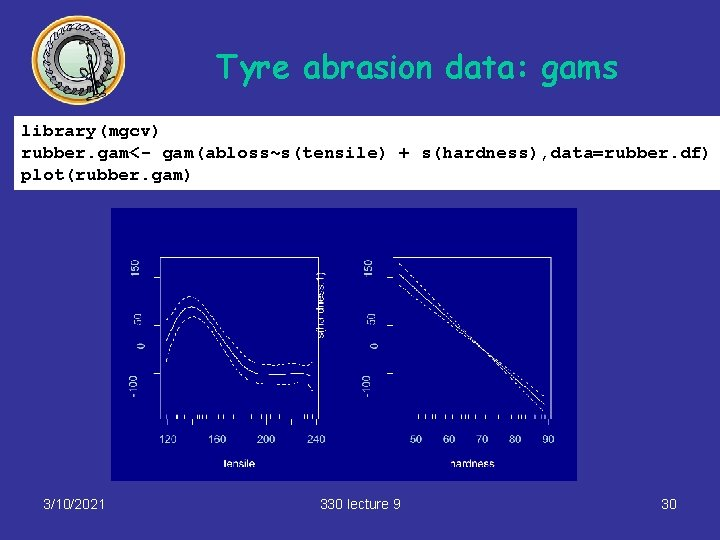Tyre abrasion data: gams library(mgcv) rubber. gam<- gam(abloss~s(tensile) + s(hardness), data=rubber. df) plot(rubber. gam) 3/10/2021 330 lecture 9 30Tyre abrasion data: Conclusions • Pairs plot : not very informative • Spinner: Hint of a “kink” • Coplot: suggestion of non-linearity, a “kink” • Residuals versus fitted values: weak suggestion that regression is not planar • gam plots: hardness is OK, but strong suggestion that tensile needs transforming • Suggested transformation: looks a bit like a cubic or 4 th degree polynomial, so try these (or a spline). Using a spline, the R 2 is increased from 84% to 94. 3%. 3/10/2021 330 lecture 9 31